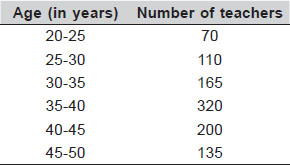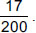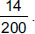# User ForumSubject :IMO    Class : Class 9

The following table gives the ages of teachers in XYZ school.₹A teacher is selected at random from the group, then which of the following statements is INCORRECT?

A Probability of a teacher having age greater than or equal to 35, isB Probability of ateacher having age more than or equal to 25 and less than 40, isC Probability of a teacher selected is of age not less than 30, isD Probability of a teacher selected from the youngest group, isIn the age group 35-40, it should be 300 instead of 320 .Only then is the answer coming ..according to the solution it is 300.

Class : Class 9
option c

Class : Class 9

Class : Class 9